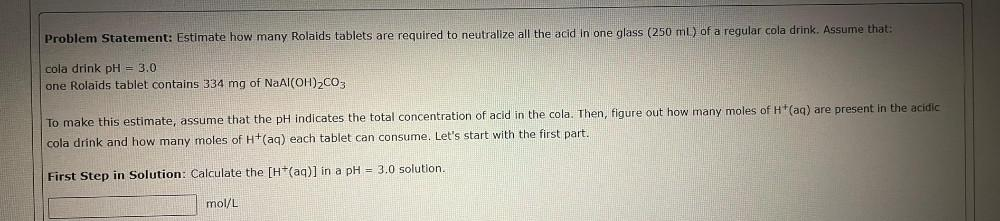Question:

# Problem Statement: Estimate how many Rolaids tablets are

Last updated: 7/25/2022Problem Statement: Estimate how many Rolaids tablets are required to neutralize all the acid in one glass (250 mL) of a regular cola drink. Assume that: cola drink pH = 3.0 one Rolaids tablet contains 334 mg of NaAl(OH)₂CO₃ To make this estimate, assume that the pH indicates the total concentration of acid in the cola. Then, figure out how many moles of H⁺(aq) are present in the acidic cola drink and how many moles of H⁺(aq) each tablet can consume. Let's start with the first part. First Step in Solution: Calculate the [H⁺(aq)] in a pH = 3.0 solution.